# Post canonical system

(diff) ← Older revision | Latest revision (diff) | Newer revision → (diff)

Post calculus

A way of defining enumerable sets (cf. Enumerable set) of words. The notion of a Post canonical system was introduced in 1943 by E. Post and was the first general notion of a calculus suitable to define arbitrary enumerable sets and not attached to the logical structure of the generated objects, to their semantics or to the logic of the derivation rules (cf. Derivation rule). A Post canonical system is given by a quadruple, whereis the alphabet of the calculus,(which has no letters in common with) is the alphabet of variables,is a list of words in(the axioms of the calculus), andis a list of derivation rules of the form(*)

(are the designations of words in;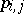are the designations of letters from). A wordis obtained from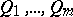by applying the rule (*) if for any letter fromin (*) one can find a word in(the value of this variable) after substitution of which at all places where the considered variable appears in (*) one obtains the wordsabove the line and the wordunder the line. On the basis of such understanding of rules a derivation is defined in the Post canonical system. In the theory of calculi one uses the following definition of an enumerable set of words in, which is equivalent to the usual one:is called enumerable if it coincides with a set of words indeduced in some Post canonical system whose alphabet contains(the necessity of the extension ofby at least one letteris non-removable but one can require that besidesonly words of the form, whereis in, should be derivable).

One can consider different specializations of the notion of a Post canonical system: 1) Post normal systems (all rules have the form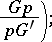2) local calculi (rules of the form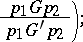3) restricted calculi (a one-letter alphabet, rules with one premise); etc.

The above-mentioned specializations are assumed to have one axiom, and an arbitrary Post canonical system can be reduced to any of them (the equivalence between a Post canonical system and a Post normal calculus (cf. Post normal system) established by Post has fundamental significance in studies in this direction in order to find unsolvable systems).

For references see Calculus.

Regular canonical systems have turned out to be of particular importance. In a regular canonical system every derivation rule is of the form "Gp yields G'p" whereand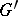are words over the alphabet of the calculus andis a variable. Further details are contained in [a1].